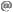# Stochastic Network Modeling (SNM) Fall 2022 Semester

Compulsary course of the Speciality Computer Networks and Distributed Systems of the Master in Innovation and Research in Informatics.

## Assessments and Final Exam Dates

• First assessment (DTMC): to be fixed
• Second assessment (CTMC): to be fixed
• Final exam (all units): 12/01/2023 11:30-14:30 (final exams period)

## Abstract:

The goal of this course is giving the student a background in stochastic processes and their application to computer networks. This is a methodological course that forms the student in mathematical stochastic modeling.

## Methodology:

4 hours per week, dedicated to magistral classes to explain the theory and solve problems. The students activities will consist of reading material and solving practical problems that will be proposed during the course.
Timetable

## Evaluation:

The theory mark will be calculated from the problems delivered by the student, assessment marks and a final exam mark. The formula for calculating the mark for the course is:
• NF = 0.1 * NP + 0.30 * max{EF, C} + 0.60 * EF
where:
• NF = final mark
• EF = final theory exam
• NP = Problems delivered by the students
• C = average assessments mark: C = 0.5*C1 + 0.5*C2

## Teacher

Llorenç Cerdà-Alabern, llorencac.upc.edu
Office: C6-213
Tel: 93.401.67.98

## Contents

1. Introduction
2. Discrete Time Markov Chains (DTMC)
3. Continuous Time Markov Chains (CTMC)
4. Queuing Theory

## References

• Numerical tools:
• Books
• Performance modeling and design of computer systems: queueing theory in action.
Harchol-Balter, Mor. Cambridge University Press, 2013.
• Probability, Stochastic Processes and Queuing Theory.
R. Nelson. Spinger, 1995.
• Introduction to probability models.
Sheldon M. Ross. Academic Press, New York, 2003
• Probability and Statistics with Reliability, Queuing, and Computer Science Applications
Kishor S. Trivedi, John Wiley and Sons, New York, 2001.
• Finite Markov Chains
John G Kemeny, J Laurie Snell. Springer, 1960.
• An Introduction to Probability Theory and Its Applications, Vol. 1, 3rd Edition
William Feller. Wiley, 1968